### Home > PC > Chapter 8 > Lesson 8.1.3 > Problem8-47

8-47.
1.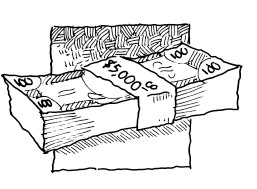Most investments receive compound interest as opposed to simple interest. This allows an investment to earn interest on the interest already received. Miranda plans to invest \$5000. Find the value of the investment after one year, given the following rates: Homework Help ✎

1. Annual interest of 12% compounded monthly. Hint: See part (d) of the previous problem.

2. Annual interest of 8% compounded quarterly (4 times per year).

3. Annual interest of 5.2% compounded weekly.

4. Annual interest of 7.3% compounded daily.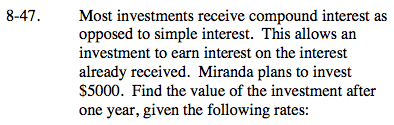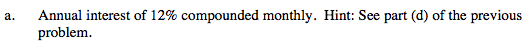y = 5000(1.01)12
y = 5,634.13 dollars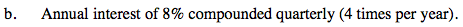y = 5000(1.02)4
y = 5,412.16 dollars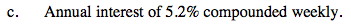Follow the same thinking process as in the parts above.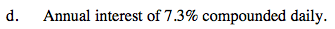Follow the same thinking process as in the part (a) and (b) above.